# Test: Alligation or Mixture- 1

## 10 Questions MCQ Test Quantitative Reasoning for GMAT | Test: Alligation or Mixture- 1

Description
Attempt Test: Alligation or Mixture- 1 | 10 questions in 20 minutes | Mock test for Banking Exams preparation | Free important questions MCQ to study Quantitative Reasoning for GMAT for Banking Exams Exam | Download free PDF with solutions
QUESTION: 1

### 8 litres are drawn from a cask full of wine and is then filled with water. This operation is performed three more times. The ratio of the quantity of wine now left in cask to that of the water is 16:65. How much wine did the cask originally hold?

Solution: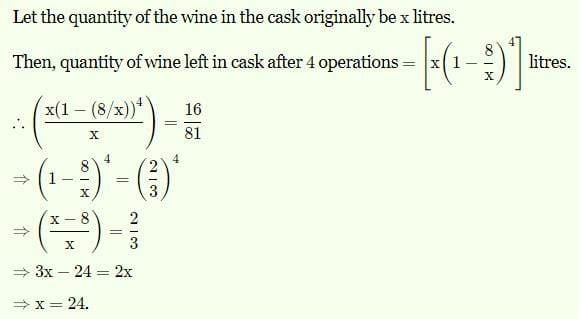QUESTION: 2

### A container contains 40 litres of milk. From this container 4 litres of milk was taken out and replaced by water. This process was repeated further two times. How much milk is now contained by the container?

Solution:

Assume that a container contains x of liquid from which y units are taken out and replaced by water. After n operations, the quantity of pure liquid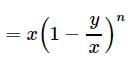Hence milk now contained by the container = 40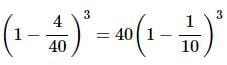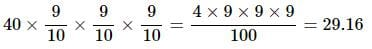QUESTION: 3

### How many kilograms of sugar costing Rs. 9 per kg must be mixed with 27 kg of sugar costing Rs. 7 per Kg so that there may be a gain of 10 % by selling the mixture at Rs. 9.24 per Kg ?

Solution:

► S.P. of 1 kg of mixture = Rs. 9.24, Gain 10%.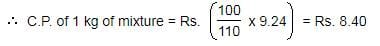By the rule of allilation, we have: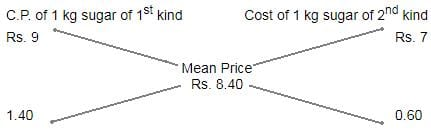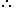Ratio of quantities of 1st and 2nd kind = 14 : 6 = 7 : 3.

► Let x kg of sugar of 1st be mixed with 27 kg of 2nd kind.

Then, 7 : 3 = x : 27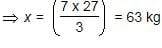QUESTION: 4

A milk vendor has 2 cans of milk. The first contains 25% water and the rest milk. The second contains 50% water. How much milk should he mix from each of the containers so as to get 12 litres of milk such that the ratio of water to milk is 3 : 5?

Solution:

► Let x and (12-x) litres of milk be mixed from the first and second container respectively

► Amount of milk in x litres of the the first container = .75x

► Amount of water in x litres of the the first container = .25x

► Amount of milk in (12-x) litres of the the second container = .5(12-x)

► Amount of water in (12-x) litres of the the second container = .5(12-x)

► Ratio of water to milk = [.25x + .5(12-x)] : [.75x + .5(12-x)] = 3 : 5

⇒ (.25x+6-5x)/(.75x+6-.5x) =3/5

⇒(6−.25x)/(.25x+6) =3/5

⇒30−1.25x=.75x+18

⇒2x=12

⇒x=6

► Since x = 6, 12-x = 12-6 = 6

Hence 6 and 6 litres of milk should mixed from the first and second container respectively

QUESTION: 5

Two vessels A and B contain spirit and water in the ratio 5 : 2 and 7 : 6 respectively. Find the ratio in which these mixture be mixed to obtain a new mixture in vessel C containing spirit and water in the ration 8 : 5 ?

Solution:

► Spirit in 1 litre mix of A = 5/7 litre.
► Spirit in 1 litre mix of B = 7/13 litre.
► Spirit in 1 litre mix of C = 8/13 litre.
By rule of alligation we have required ratio X:Y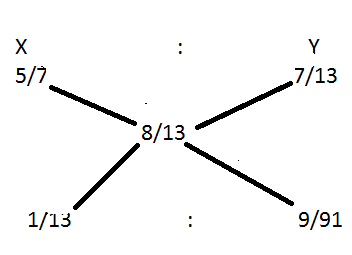Therefore required ratio = 1/13 : 9/91
= 7:9

QUESTION: 6

Tea worth Rs. 126 per kg and Rs. 135 per kg are mixed with a third variety of tea in the ratio 1 : 1 : 2. If the mixture is worth Rs. 153 per kg, what is the price of the third variety per kg ?

Solution:

► Since first and second varieties are mixed in equal proportions.So, their average price = Rs.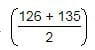= Rs. 130.50

► So, the mixture is formed by mixing two varieties, one at Rs. 130.50 per kg and the other at say, Rs. x per kg in the ratio 2 : 2, i.e., 1 : 1. We have to find x.

By the rule of alligation, we have: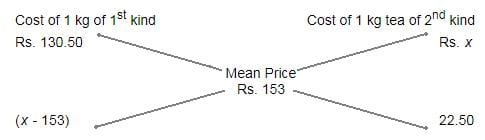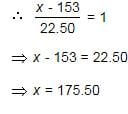QUESTION: 7

A jar is full of whiskey contains 40% alcohol. A part of this whiskey is replaced by another containing 19% alcohols and now the percentage of alcohol was found to be 26%. The quantity of whiskey replaced is:

Solution:

By the rule of alligation we have
► So ratio of 1st and 2nd quantities = 7:14
= 1:2
∴ Required quantity replaced = 2/3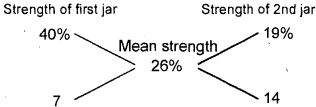QUESTION: 8

The cost of Type 1 material is Rs. 15 per kg and Type 2 material is Rs.20 per kg. If both Type 1 and Type 2 are mixed in the ratio of 2 : 3, then what is the price per kg of the mixed variety of material?

Solution:

► Cost Price(CP) of Type 1 material is Rs. 15 per kg
► Cost Price(CP) of Type 2 material is Rs. 20 per kg
Type 1 and Type 2 are mixed in the ratio of 2 : 3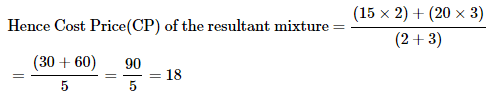Therefore, rice per kg of the mixed variety of material = Rs.18

QUESTION: 9

Find the ratio in which rice at Rs. 7.20 a kg be mixed with rice at Rs. 5.70 a kg to produce a mixture worth Rs. 6.30 a kg.

Solution: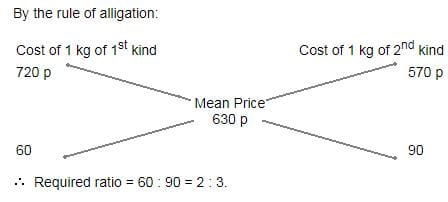QUESTION: 10

In what ratio should rice at Rs.9.30 per Kg be mixed with rice at Rs. 10.80 per Kg so that the mixture be worth Rs.10 per Kg?

Solution: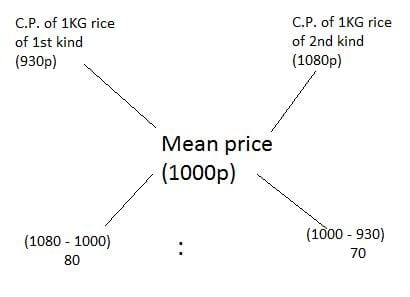Thus, required ratio = 80 : 70 = 8 : 7Use Code STAYHOME200 and get INR 200 additional OFF Use Coupon Code